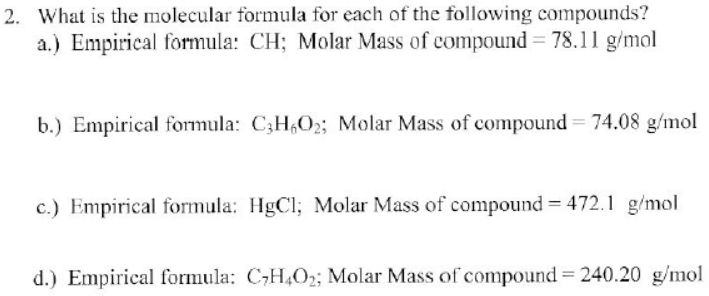# Problem: What is the molecular formula for each of the following compounds? (a) Empirical formula: CH: Molar Mass of compound = 78.11 g/mol (b) Empirical formula: C3H6O2: Molar Mass of compound = 74.08 g/mol (c) Empirical formula: HgCl: Molar Mass of compound = 472.1 g/mol(d) Empirical formula: C7H4O2: Molar Mass of compound = 240.20 g/mol

###### FREE Expert Solution
100% (159 ratings)###### Problem Details

What is the molecular formula for each of the following compounds?

(a) Empirical formula: CH: Molar Mass of compound = 78.11 g/mol

(b) Empirical formula: C3H6O2: Molar Mass of compound = 74.08 g/mol

(c) Empirical formula: HgCl: Molar Mass of compound = 472.1 g/mol

(d) Empirical formula: C7H4O2: Molar Mass of compound = 240.20 g/mol